### CHAPTER 7. MAGNITUDE AND ORIENTATION OF ANGULAR MOMENTUM PROPERTIES OF THE EVOLUTION OF DOUBLE GALAXIES

Quantitative data about the magnitude of the orbital and internal (spin) angular momenta of double galaxies, their relationship and relative orientation, have exceptional value from the point of view of the cosmogony of these simpler systems of galaxies. The study of angular momenta in pairs has received relatively little recent attention. Within the history of astronomy, there have been several examples of the way in which the problem of the systemic angular momentum (planets, stars, and so forth) has been of unique value in testing any cosmogonic hypothesis.

Karachentsev (1981d, e) and White et al. (1983) showed that the orbital motion of double galaxies is near circular, with a typical eccentricity e = 0.25 (Karachentsev, 1985). It follows that pairs of galaxies possess a large orbital angular momentum. The preeminence of circular motion for double galaxies appears to be reasonably well established. However, the numerical experiments of Turner et al. (1979) established that pairs formed by gravitational clustering should have highly elongated orbits with eccentricity e0.7 - 0.9. Another mechanism which might form double galaxies is the evolution of systems of galaxies of higher multiplicity. Anosova (1969) and later Nash and Monaghan (1978) dynamically modelled the dissociation of triplets, and found that the distribution of the pair's orbital eccentricity after the departure of the third component has a maximum at e = 1. Therefore circular motion must be quite atypical in both of these formation scenarios.

Recently, White (1978) and other authors have conducted computer modelling of the effects of dynamical friction on close pairs of galaxies. Double galaxies which approach each other within distances comparable to the size of their outer regions should merge into single systems in a time on the order of a few orbital periods. In considering dynamical friction, the further evolution of a double galaxy will depend strongly on its orbital eccentricity. The longest lasting systems will be those with circular motions. Energy loss and merging of double galaxies lead to an evolutionary selection effect which possibly accounts for the observed circular motions of pairs which have survived to the present epoch.

Consider the observational material on the angular momenta of double galaxies. The first estimates were made by Gorbachov (1970a). For 437 pairs selected from the MCG catalogue on the basis of structural signs of interaction, Gorbachov found that the orbital angular momentum of double galaxies is greater than the sum of their internal angular momenta by a mean factor of 1.2 to 1.6. A limitation of this work is the ill-defined selection criterion and the resultant non-uniformity of the sample. The distribution of 239 SS pairs by orbital and spin angular momentum from a uniform (but not very deep) subsample was presented by Karachentsev (1981f). We can now study complete data for the 487 isolated pairs in our catalogue.

Table 28.

Assuming a uniform rotating spheroid (Zasov and Ozernoi, 1967) the total angular momentum of the galaxy may be expressed simply as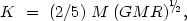(7.1)

where M and R are the mass and radius of the galaxy and G is the gravitational constant. In order to use (7.1) we must know the exact radius of the galaxy. One must also take into account that not all of the mass of the galaxy (especially for early types) rotates at the velocity characteristic of the disk subsystem. In calculating the total internal angular momentum for pair members we took the radius of the galaxy to the standard isophote 25m / sq.arc sec., i.e., R = A25 / 2, and the mass was derived from the integrated luminosity, M = fL. We used the mass-to-luminosity ratio f for each structural type as shown in the first line of table 28. The second line is the dimensionless factorTy = (Md / M)(Ld / L) which characterizes the fraction of the mass (luminosity) located in a thin subsystem (disk). The correction coefficientsTy were calculated for various systems using the data of Yoshizawa and Wakamatsu (1985) and Grosbol (1985). For elliptical galaxies we choseE = 0.20 based on the fact that the globular clusters in the Galaxy have a systematic rotation five times slower than the disk stars. With these extra relations, (7.1) becomes (11)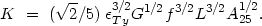(7.2)

For a unit we have adopted the angular momentum of our own Galaxy KG = 0.97 × 1074 g × cm2 / s, calculated from (7.2) for MG = 1.5 × 1011 M, AG = 30 kpc, andSb = 0.9.

The distribution of the total angular momentum in double galaxies in units of KG is shown in figure 47, divided by morphological type. The mean value and its standard deviation are shown in the third line of table 28. The total angular momentum increases on going from types E and S0 to Sa , and then drops rapidly for much later types. The mean value of the entire subsample of 974 components is <K> = 1.63 ± 0.13 with standard deviation(K) = 2.90.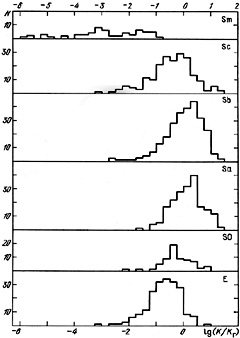Figure 47.

In collecting the individual mass estimates for 209 pair members (Karachentsev, 1985) we determined the angular momentum of these galaxies directly from the values A25 and M(A25) for the same structure parametersTy. The mean values are shown in the fourth line of table 28. These angular momenta exhibit an analogous trend along the Hubble sequence with a maximum at type Sa. The differences in <K> between these two subsamples arise from the variation of the individual values f = M/L among galaxies, and also from selection effects, since the mass estimates have usually been performed for the very nearest objects, which have luminosities and masses below the catalogue mean.

The bivariant distribution of double systems according to the angular momenta of their components shows them to be tightly coupled. Most of the effect arises from the correlation of structural types among pair members and the dependence of the angular momentum on the Hubble type. To exclude this possibility we calculate the correlation coefficients for each subsample broken down by structural type, and obtain +0.66 (E E), +0.56 (Sa Sa), +0.44 (Sb Sb), +0.15 (Sc Sc) and +0.92 (Sm Sm). Therefore there is a stronger mutual dependence between the spin values than can be explained by the known correlation between Hubble types.

In addition to the total angular momentum, another important characteristic is the specific angular momentum k = K / M expressed per unit mass. The mean values of k for 209 double galaxies of various types are given in the last line of table 28. We have adopted the spin of our Galaxy as the unit of measurement: kG = 3.24 × 1029 cm2 / s. As might be expected, double galaxies vary less in their specific, than in their total, angular momentum. The maximum specific angular momentum is characteristic of spiral galaxies of type Sa. This is not significantly different from the conclusions of Dai Wen-sai et al. (1979), who studied the distribution of specific angular momentum for 183 spiral galaxies of various types and found a maximum at type Sb.

Dai Wen-sai et al. (1979) noted a relation between the specific spin for spiral galaxies and their mass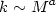(7.3)

with index a = 2/3. Carrasco et al. (1982) extended (7.3) to a wide variety of celestial objects from asteroids and double stars to superclusters, finding that the index a is always within the interval (2/3 to 3/4), which reflects the rotational stability of gravitationally-bound systems to a so-called `gyro-catastrophe'.

For double galaxies with individual estimates of M the distribution of specific angular momentum and mass is shown in figure 48. The filled dots indicate data for Sc spirals, the crosses for Sm, and the open circles for ellipticals. Objects of each type are distributed in narrow zones with a scatter(log k)0.07. The difference in specific angular momentum (for unit mass) for Sc and E galaxies agrees with the difference in the structure parameterTy. The observed data for Sc and Sm galaxies suggests a common sequence. The behaviour of k(M) for Sb, Sa and S0 types (not shown on the figure) is analogous, at smaller values of the specific angular momentum.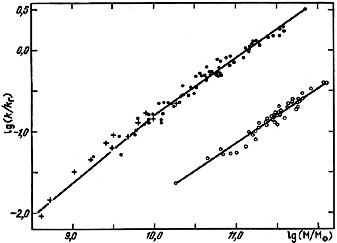Figure 48.

In the region log(M / M) > 10, the specific angular momentum/mass relation for galaxies of each morphological type is well represented by (7.3) with index a = 7/10. For dwarf galaxies, this dependence has a somewhat steeper slope, a = 5/6. These respective relations are shown as straight lines in figure 48. The distributions of double galaxies in log k and log M, partly reflected in the values for the index a in (7.3), indicate that the relation between log M and log A25 has a break slightly below log M = 10. For objects of large mass it follows that M ~ A255/2, while for dwarf galaxies it is flatter: M ~ A253/2. Inserting these expressions into k ~ (MA25)1/2 gives values for the index a which will be given below. We note that the structural dependencies of angular momentum on mass, diameter, morphological type, or even on differences between field objects and system members, have been virtually unstudied until now, and therefore any questions about possible differences between double galaxies and single systems or group members are in real doubt.

We now examine the data on the orbital angular momenta of double galaxies. Two points with masses M1 and M2 in circular Keplerian motion have an absolute value for their total orbital angular momentum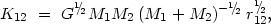(7.4)

where r12 is the spatial separation between centers. In calculating the orbital angular momentum for pairs we estimate the component masses from the luminosity, M = fL, using individual values of the mass-to-luminosity ratio f for each morphological type, as shown in table 28. The spatial separation is estimated from the observed projected separation X accounting for systematic underestimates of orbital momentum with a mean factor (72)1/2 /2(1/4)0.874. The distribution of the absolute value of the total angular momentum for 487 catalogue pairs is shown in figure 49 on a logarithmic scale. As for the internal angular momentum, the units of measurement are taken to be the total spin of our Galaxy KG. The range of values is quite large, covering nearly eight orders of magnitude. Low values of K12 occur only for pairs with one or two components of type Sm, which have small masses. These systems are shown as the cross-hatched regions. About three quarters of the pairs have orbital angular momenta exceeding the angular momentum of our Galaxy, and about 20% of the subsample exceed KG by more than an order of magnitude. The mean value of K12 for all double systems is 6.73KG, with standard deviation 10.5KG.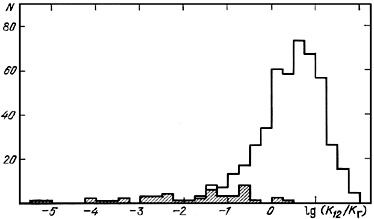Figure 49.

Pairs containing galaxies of different morphological types have systematic differences in luminosity and mass of the components as well as in their characteristic separation. This propagates to differences in the orbital angular momentum depending on the Hubble types of the component galaxies. The mean values for pairs with various types of component, and the standard error of the mean, are shown in the upper lines of table 29. These results show a decrease by a factor of 2 or 3 from E to Sc and a sharp drop for pairs with Sm galaxies.

Table 29.

Besides the importance of the orbital and individual angular momenta, there is independent interest in their dimensionless ratio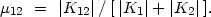(7.5)

Figure 50 shows the distribution of this ratio. The lower part shows pairs with spiral components, with cross-hatching indicating pairs consisting of Sm galaxies. Mixed doubles (SE) are shown in the middle section, and pairs with elliptical galaxies on the top. As expected, systems containing one or two elliptical galaxies exhibit high values of the ratio of momenta, by a factor of several compared to the other systems. The mean values of this ratio and its dependence on morphological type are shown in the lower lines of table 29. The maximum value µ1210 is shown by EE pairs, the components of which rotate slowly. Most double systems, excluding Sm galaxies, lie in the range µ12 < 1. Pairs with only spiral components (S0 - Sc) are bounded in the rather narrow interval 0.4 < µ12 < 4: depending on the Hubble subtype the mean value changes from 1.3 to 2.5 (12) . Thus, pairs of spiral galaxies have orbital angular momenta of the same order of magnitude as the sum of the internal angular momenta, but in EE or ES systems the basic rotational momenta are overwhelmed by the orbital motion.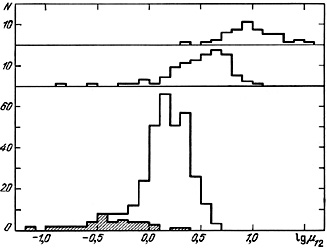Figure 50.

We note several circumstances affecting the distribution of the ratio of rotational momenta for double galaxies. After incorporating various galactic parameters, (7.5) becomes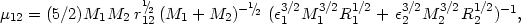(7.6)

where Ri is the galaxy radius and the structure factori incorporates the fraction of the mass of a galaxy of a given type participating in the internal rotation. Introducing the dimensionless parametersm = M2 / M1 andR = R2 / R1 and expressing the separation in units of the radius of the primary component,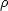= r12 / R1, (7.6) becomes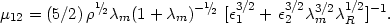(7.7)

A further simplification may be obtained by incorporating an average relation between mass and radius, M ~ R2, and adopting1 =2 = 1. In this case there is an obvious dependence of the ratio of momenta on the geometric factors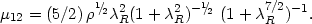(7.8)

It is apparent from this that pairs of identical galaxies in contact should have a ratio µ12 = 5/4.

The calculation of the orbital angular momenta of double galaxies assumed purely circular orbits. In the general case of elliptical motion with eccentricity e the orbital momentum is reduced to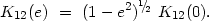(7.9)

For the typical eccentricity e = 0.25 (see section 4.4) the correction is only 3%.

Finally, we must note that the above data on the orbital and angular momenta are for the catalogue sample of double galaxies. There are noticeable differences between the overall spatial distribution and the catalogue distribution, due to selection effects. Specifically, the isolation criteria lead to a strong exclusion of wide pairs. While the mean separation between catalogue double galaxies is 38.0 kpc, by contrast per unit volume this value is 65.1 kpc (section 3.4). Taking this into account increases the orbital angular momenta by (65.1 / 38)1/21.3 times.

Our last remark deals with various techniques for calculating the orbital angular momentum of pairs. Recent work by Verner (1985) proposes using estimates of the orbital mass M = (32 / 3) G-1 y2X to estimate K12. This approach allows the orbital momentum to be calculated from the immediate observed quantities y and X. However, because of the projection factors, K12 estimated by this method will exhibit strong fluctuations about the true value K120. The situation is actually even worse, since it is impossible to neglect the role of radial velocity measurement errors in arriving at statistically independent estimates of the true orbital momentum. For small errors the distribution p (K120) is easy to derive from the observed p (K12) by standard methods such as the method of statistical moments.

11 The angular momenta were determined by a very simple scheme. The actual situation may be much more complex, if the mass and luminosity of galaxies are differently distributed, or the rotational velocity for stars in disks does not agree with estimates based on the motion of gas in disks. Back.

12 In the class S0-S0 there is only a single pair. Back.